# MAP Function in Google Sheets – LAMBDA Helper Function

The MAP function in Google Sheets creates an array of data from an input range, where each value is “mapped” to a new value based on a custom LAMBDA function.

MAP takes an input range of data from your Sheet, then performs an operation on each value of that range, and puts the results into an output array of the same dimensions as the original array.

Let’s see a silly example to understand how the MAP function works.

We’re going to transform an array of data into thumbs up for values 5 and over, and skull and crossbones for values less than 5. Stay with me here 😉

Here’s the input array: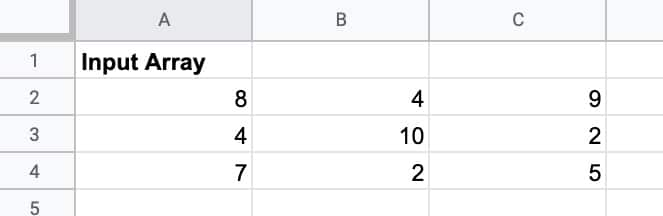To which we apply this MAP formula:

`=MAP(A2:C4, LAMBDA(value, IF(value >= 5 , "👍" , "☠️" )))`

MAP passes each value to the LAMBDA, which uses a regular IF function to test if the value is greater than or equal to 5, and output a thumbs up or skull.

To give an output array of: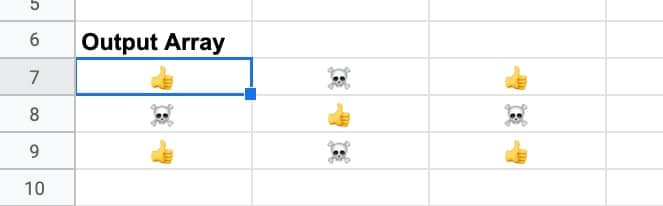If I highlight the values, you can see how MAP evaluates each value against the test, and transforms it into a thumbs up or a skull and crossbones.

The 9 has mapped to a thumbs up, and the 2 has mapped to a skull and crossbones.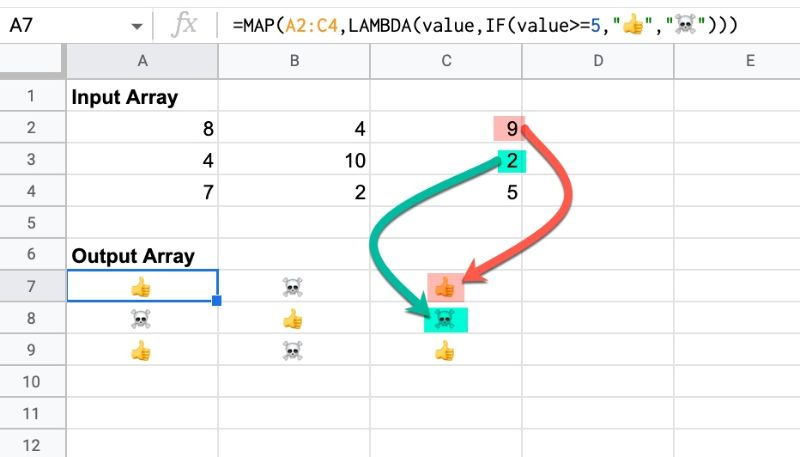Ok, so this isn’t a particularly useful example, but it’s a nice illustration of how the MAP function works.

There are some more practical examples further down this post.

🔗 Get this example and others in the template at the bottom of this article.

## MAP Function Syntax

`=MAP(array1, [array2, …], lambda)`

It takes 2 or more arguments:

`array1`

This is the first input array that you want to map into a new array.

`[array2, …]`

These are optional, additional input arrays to use in the map operation.

`lambda`

This is the function operation that will be performed on each item in the input array. The LAMBDA function must have a placeholder variable for each input array.

### MAP Function Notes

• The position of items in the array is not changed by the MAP function. So the item at position (1,1) will map to position (1,1).
• MAP will work with array literal intputs also. See the simple example that follows.
• The MAP function in Google Sheets accepts multiple input arrays. The input arrays must have the same dimesions. Each input array requires a variable in the lambda expression.

## MAP With Array Literal Inputs

The MAP function accepts array literal inputs, which are arrays created with curly brackets {…}.

For example, this formula uses a simple LAMBDA function to double each number in the input array literal. The output in this case is another array: 2, 4, 6

=MAP({1,2,3},LAMBDA(x, x*2))

The MAP function passes each number into the LAMBDA in turn, so 1 doubles to 2, 2 doubles to 4, and 3 doubles to 6. The results form a new output array of the same dimension.

## MAP Formula Temperature Example

Suppose you have this dataset, which displays the average annual high and low temperatures for places in England: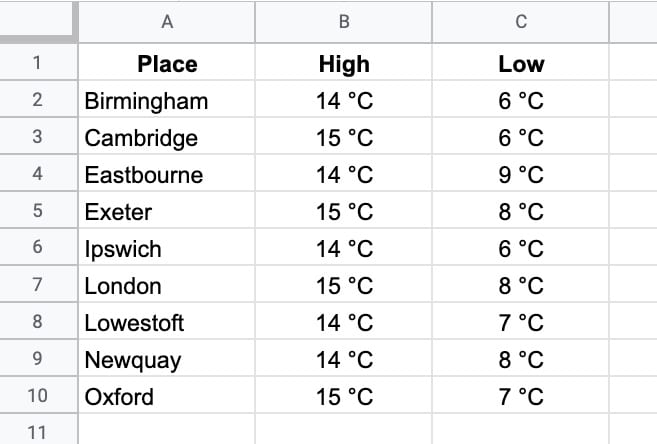Let’s use MAP to convert them to an array of temperatures in Fahrenheit.

You could do this with regular formulas, or array formulas, but this kind of array transformation is ideal for the MAP function. You can do the transformation with a single formula, without needing an array formula.

Let’s see how.

To begin, we’ll use a REGEXEXTRACT function to extract the digits (and drop the °C), where t is the placeholder variable representing the input temperature:

`REGEXEXTRACT(t,"\d+")`

Then we’ll convert the celsius temperature to a Fahrenheit temperature with the CONVERT function:

`CONVERT(REGEXEXTRACT(t,"\d+"),"C","F")`

Then we’ll round that with the ROUND function and append the °F to indicate Fahrenheit:

`ROUND(CONVERT(REGEXEXTRACT(t,"\d+"),"C","F"))&" °F"`

This is the basis for our LAMBDA function:

`LAMBDA(t,ROUND(CONVERT(REGEXEXTRACT(t,"\d+"),"C","F"))&" °F")`

Finally, we can complete the MAP formula:

`=MAP(B2:C10, LAMBDA(t, ROUND( CONVERT( REGEXEXTRACT(t,"\d+"),"C","F"))&" °F"))`

This single MAP formula creates a new array by transforming each element of the original array.

The output looks like this: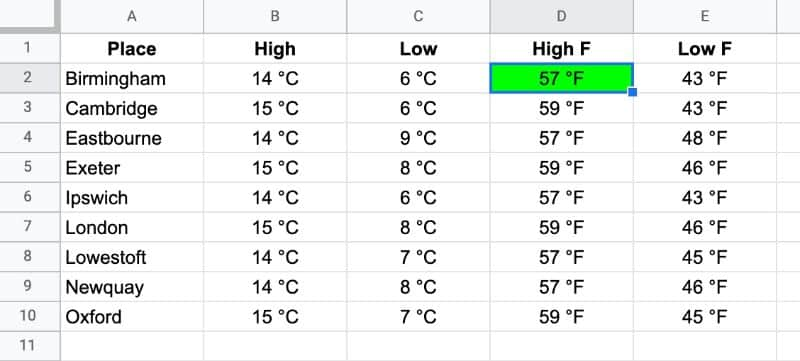And here’s a visual explanation of how it works: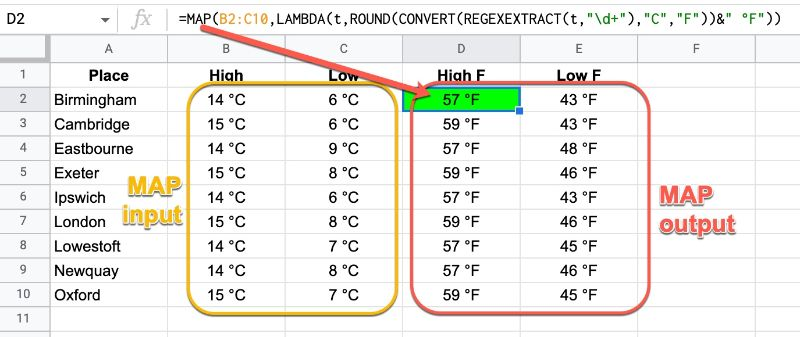## Multiple Input Arrays

MAP also accepts multiple input arrays.

For example, this formula calculates the max value at a given position from two input arrays.

`=MAP(A1:C3,E1:G3,LAMBDA(a,b,MAX(a,b)))`

Here, in the lambda, “a” represents the value from the first input array, and “b” represents the value from that same position (e.g. 1,1) of the second input array.

If I had a third input array, I would need another variable (e.g. “c”) in my lambda expression.

The returned array consists of all the max values.

## MAP Function Template

Feel free to make a copy: File > Make a copy…

If you can’t access the template, it might be because of your organization’s Google Workspace settings.

In this case, right-click the link to open it in an Incognito window to view it.

See the LAMBDA function and other helper functions MAP, REDUCE, MAKEARRAY, etc. here:

Named Functions and New Functions In Google Sheets For 2022

## 5 thoughts on “MAP Function in Google Sheets – LAMBDA Helper Function”

1.Sanchit Aggarwal says:

Hey Ben,
The MAP function in Sheets now accepts multiple input arrays.

Cheers,
Sanchit

1.Ben says:

Thanks for the heads up, Sanchit! I’ve updated the article to reflect that.

1.Andy says:

Hi Ben
Is there a way to handle the full input array within the lambda function, rather than an element at a time? Imagine I wanted to pass in some data, then map each value to its distance from the average of the data set…

If I just need to finish the 10 day course then please feel free to tell me, and thanks for looking!

Thanks
Andy

1.Ben says:

Hey Andy,

Here’s a way to do it with the BYROW function:

`=BYROW(B2:B7,LAMBDA(r,r-AVERAGE(B2:B7)))`

Don’t know a way to do it with the map function, with the full input array as you asked though.

Cheers,
Ben

2.Vikram Hattangady says:

Maybe this is too late to be helpful, but this works:
=MAP(B2:B7,LAMBDA(input,input-AVERAGE(\$B\$2:\$B\$7)))

If the word ‘dataSet’ is important for debugging or ease of understanding, you could wrap it in a LET formula
=LET(dataSet,\$B\$2:\$B\$7,MAP(B2:B7,LAMBDA(input,input-AVERAGE(dataSet))))
going 1 set further and giving names to both ranges,
=LET(inputRange,B2:B7,dataSet,\$B\$2:\$B\$7,MAP(inputRange,LAMBDA(input,input-AVERAGE(dataSet))))